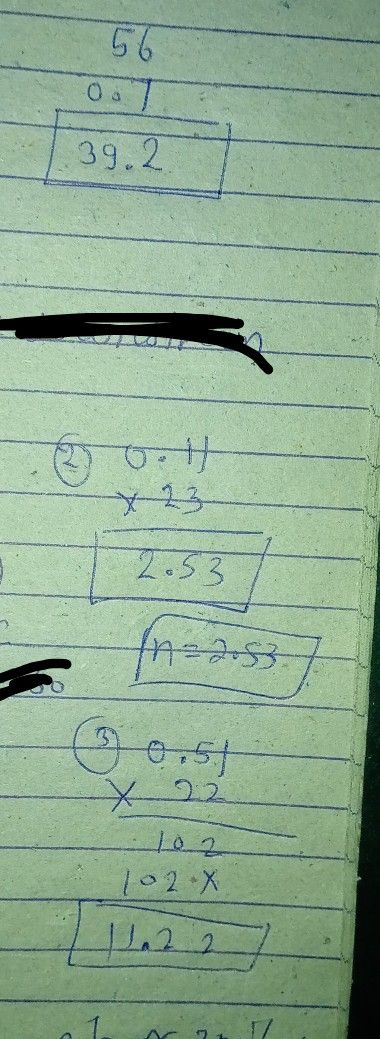Symbol
ProblemIt's time check what you've learned from the previous examples. $I.$ Answer the following questions. $1.$ What is the product of $56$ and $0.77$ $2$ What is the value of n in the equation $0.11\times 23=$ n? $3.$ What is $0.51$ multiplied by $222$ $4.$ What is $1.5$ times $0.$ 0.4? $5.$ What is the product of $0.02$ and $347$
Other
SolutionQanda teacher - Rashmi kawe are only one question allow at time by qanda policy
if you have more questions so pls ask me preferred match on my profile
thanksStudent
thankyou?maamQanda teacher - Rashmi ka
welcome
can you please evaluate ans with gift coins and also rating 5 star